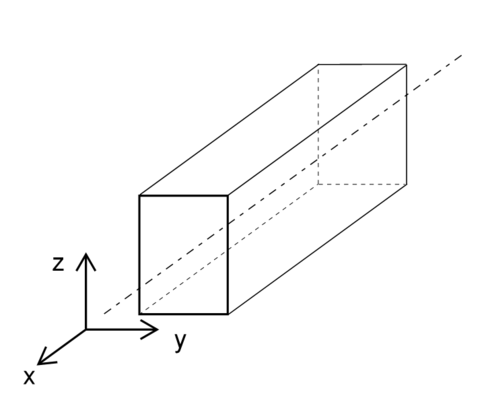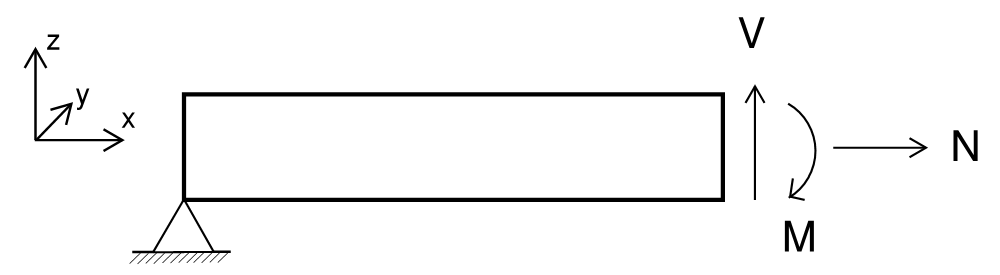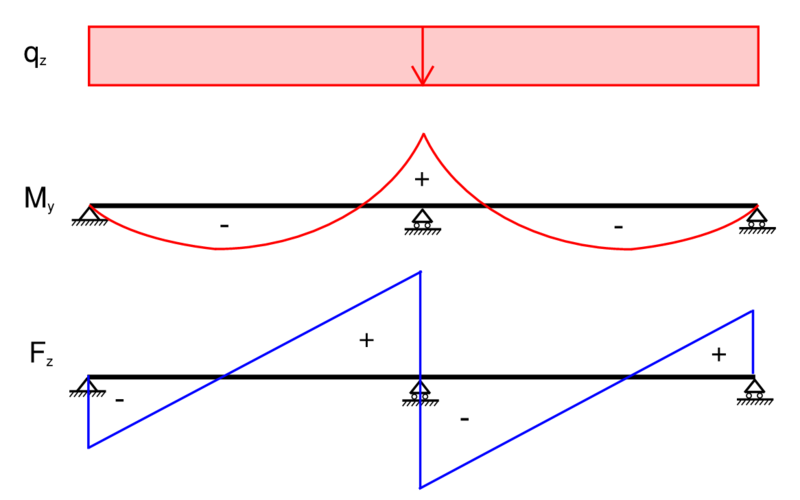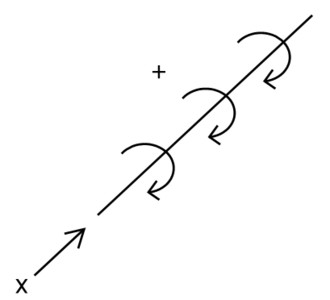# Structural and geometrical conventions

## 1D-elements

### Coordinate system

The following local coordinate system is adopted for 1D-elements e.g. beams, columns etc:• x-axis along the centre line of the element from start to end
• z-axis as the normal direction of the element
• y-axis transverse to the normal

Linear elements

For non-vertical members the local z is aligned with the global z and rotated with the orientation angle around the local x.

For vertical members the local y is aligned with the global y and rotated with the orientation angle around the local x.

A bar is vertical if its projected length to the horizontal plane is less than 0.0001, i.e. a tolerance of 0.1mm on verticality.

Curved planar elements

For curved elements the local z is aligned with the normal of the plane that the curve fits in and rotated around the curve axis with the orientation angle.

### Section property nomenclature

Area - Area of the section property
Iy - Second moment of area, major axis
Iz - Second moment of area, minor axis
Wel,y - Elastic bending capacity, major axis
Wel,z - Elastic bending capacity, minor axis
Wpl,y - Plastic bending capacity, major axis
Wpl,z - Plastic bending capacity, minor axis
Rg,y - Radius of gyration, major axis
Rg,z - Radius of gyration, minor axis

Vz - Distance centre to top fibre
Vp,z - Distance centre to bottom fibre
Vy - Distance centre to rightmost fibre
Vp,y - Distance centre to leftmost fibre
As,z - Shear area, major axis
As,y - Shear area, minor axis

### Signs of section forces

The directions for the section forces in a cut of a beam can be seen in the image below:This is: * Normal force positive along the local x-axis * Shear forces positive along the local y and z-axes * Bending moments positive around the local axis by using the right hand rule

#### Axial force Fx

Positive (+) = Tension
Negative (-) = Compression

#### Major axis bending moment My and shear force Fz

As shown in the following diagram.#### Minor axis bending moment Mz and shear force Fy

Same sign convention as for major axis.

#### Torsional moment Mx

The torsional moment follows the Right-hand rule convention.### Bar offsets

Bar offsets specify a local vector from the bars node to where the bar is calculated from, with a rigid link between the Node object and the analysis bars end point.

Hence: * a BHoM bars nodes are where it attaches to other nodes, * offsets are specified in the local coordinate system and is a translation from the node, * local x = bar.Tangent(); * local z = bar.Normal(); * node + offset is where the bar node is analytically * the space between is a rigid link

Last update: September 20, 2023